# Dijkstras Algorithm Dijkstras Algorithm This algorithm finds the

• Slides: 7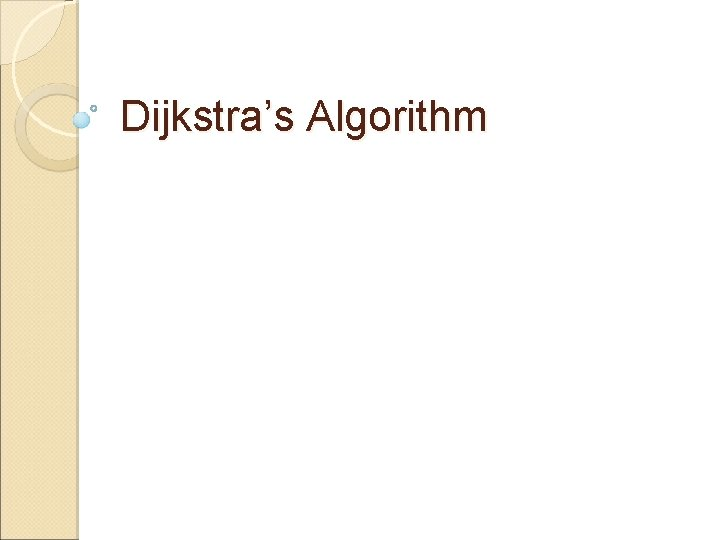Dijkstra’s AlgorithmDijkstra’s Algorithm This algorithm finds the shortest path from a source vertex to all other vertices in a weighted directed graph without negative edge weights. 5 a 10 2 3 2 b 8 3 16 4 4 12 e d c 13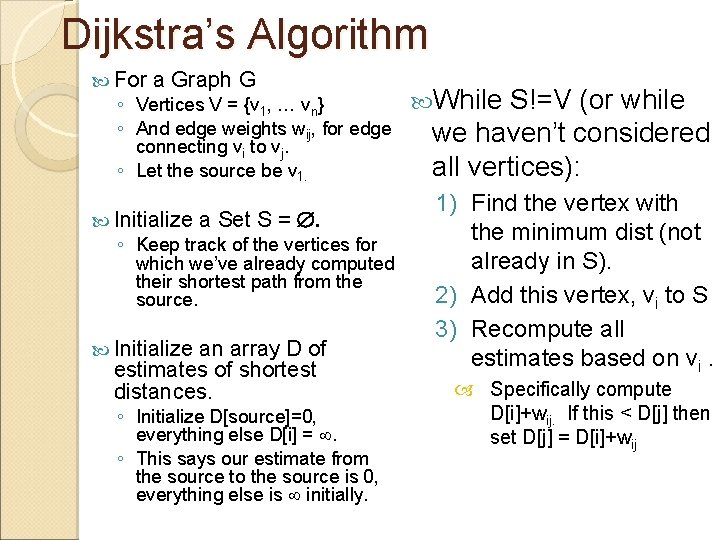Dijkstra’s Algorithm For a Graph G ◦ Vertices V = {v 1, … vn} ◦ And edge weights wij, for edge connecting vi to vj. ◦ Let the source be v 1. Initialize a Set S = . ◦ Keep track of the vertices for which we’ve already computed their shortest path from the source. Initialize an array D of estimates of shortest distances. ◦ Initialize D[source]=0, everything else D[i] = . ◦ This says our estimate from the source to the source is 0, everything else is initially. While S!=V (or while we haven’t considered all vertices): 1) Find the vertex with the minimum dist (not already in S). 2) Add this vertex, vi to S 3) Recompute all estimates based on vi. Specifically compute D[i]+wij. If this < D[j] then set D[j] = D[i]+wij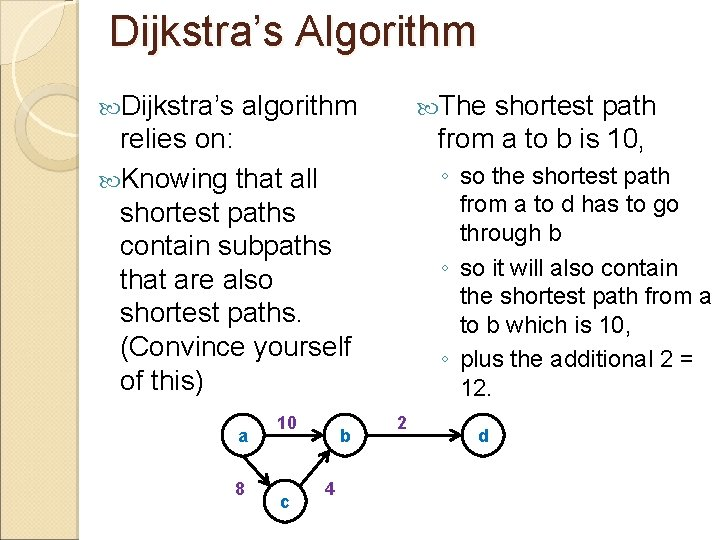Dijkstra’s Algorithm Dijkstra’s algorithm The shortest path from a to b is 10, relies on: Knowing that all shortest paths contain subpaths that are also shortest paths. (Convince yourself of this) a 8 10 c b 4 ◦ so the shortest path from a to d has to go through b ◦ so it will also contain the shortest path from a to b which is 10, ◦ plus the additional 2 = 12. 2 d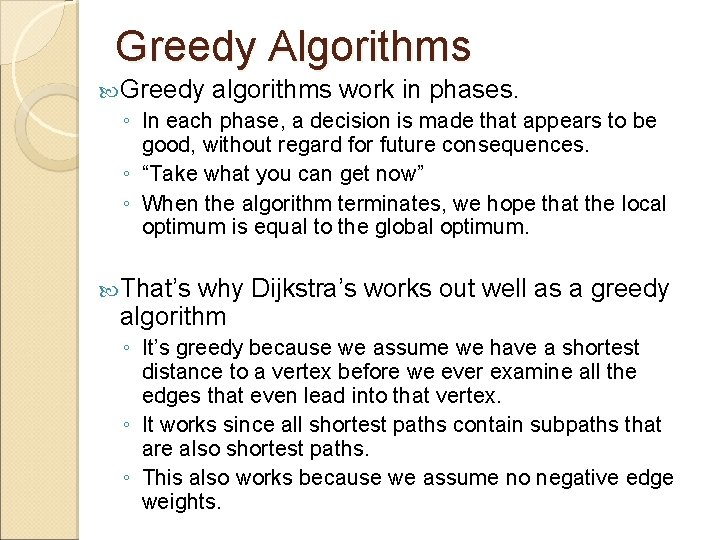Greedy Algorithms Greedy algorithms work in phases. ◦ In each phase, a decision is made that appears to be good, without regard for future consequences. ◦ “Take what you can get now” ◦ When the algorithm terminates, we hope that the local optimum is equal to the global optimum. That’s why Dijkstra’s works out well as a greedy algorithm ◦ It’s greedy because we assume we have a shortest distance to a vertex before we ever examine all the edges that even lead into that vertex. ◦ It works since all shortest paths contain subpaths that are also shortest paths. ◦ This also works because we assume no negative edge weights.Example on the board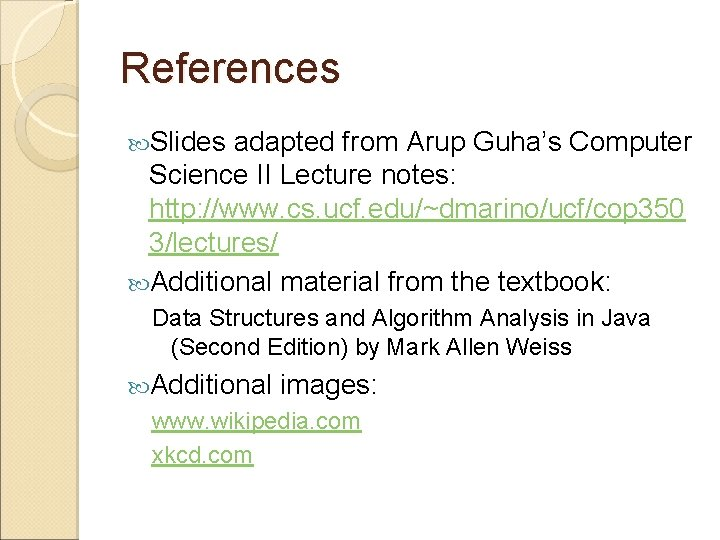References Slides adapted from Arup Guha’s Computer Science II Lecture notes: http: //www. cs. ucf. edu/~dmarino/ucf/cop 350 3/lectures/ Additional material from the textbook: Data Structures and Algorithm Analysis in Java (Second Edition) by Mark Allen Weiss Additional images: www. wikipedia. com xkcd. com